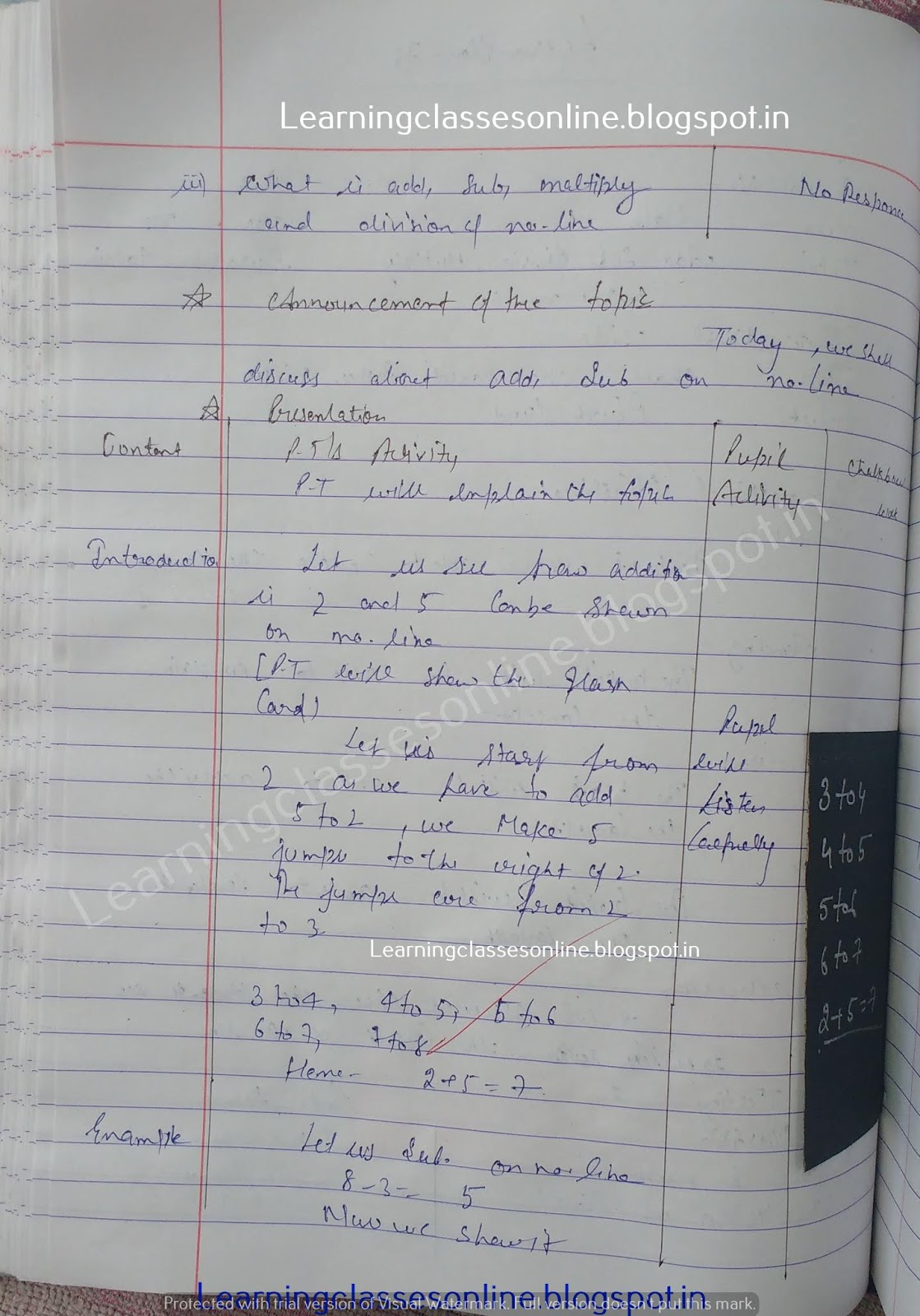Mathematics Lesson Plan on Add, subtract, division and multiply on the number line

# Mathematics Lesson Plan on Add, subtract, division and multiply on the number line

Mathematics Lesson Plan on Add, subtract, division and multiply on the number line for grade 4 to 9 teachers

Note: The Mathematics Lesson Plan given below is just an example. You can change the Name, Class, Course, Date, Duration, etc. according to your needs.